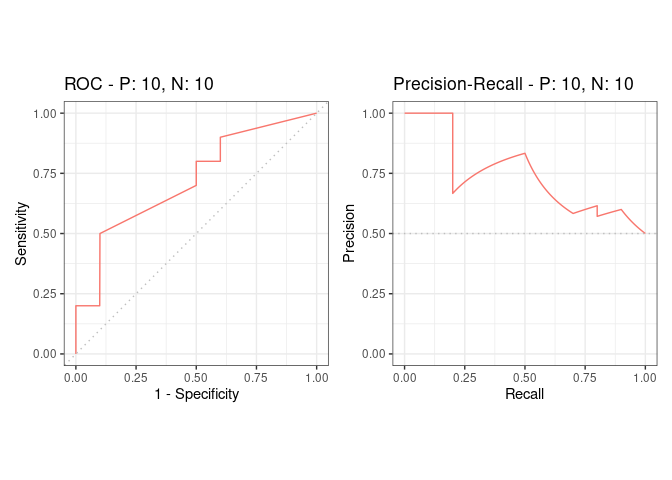# PrecrecThe aim of the `precrec` package is to provide an integrated platform that enables robust performance evaluations of binary classifiers. Specifically, `precrec` offers accurate calculations of ROC (Receiver Operator Characteristics) and precision-recall curves. All the main calculations of `precrec` are implemented with C++/Rcpp.

## Documentation

• Package website – GitHub pages that contain all precrec documentation.

• Introduction to precrec – a package vignette that contains the descriptions of the functions with several useful examples. View the vignette with `vignette("introduction", package = "precrec")` in R. The HTML version is also available on the GitHub Pages.

• Help pages – all the functions including the S3 generics except for `print` have their own help pages with plenty of examples. View the main help page with `help(package = "precrec")` in R. The HTML version is also available on the GitHub Pages.

## Six key features of precrec

### 1. Accurate curve calculations

`precrec` provides accurate precision-recall curves.

• Non-linear interpolation
• Elongation to the y-axis to estimate the first point when necessary
• Use of score-wise threshold values instead of fixed bins

`precrec` also calculates AUC scores with high accuracy.

### 2. Super fast

`precrec` calculates curves in a matter of seconds even for a fairly large dataset. It is much faster than most other tools that calculate ROC and precision-recall curves.

### 3. Various evaluation metrics

In addition to precision-recall and ROC curves, `precrec` offers basic evaluation measures.

• Error rate
• Accuracy
• Specificity
• Sensitivity, true positive rate (TPR), recall
• Precision, positive predictive value (PPV)
• Matthews correlation coefficient
• F-score

### 4. Confidence interval band

`precrec` calculates confidence intervals when multiple test sets are given. It automatically shows confidence bands about the averaged curve in the corresponding plot.

### 5. Calculation of partial AUCs and visualization of partial curves

`precrec` calculates partial AUCs for specified x and y ranges. It can also draw partial ROC and precision-recall curves for the specified ranges.

### 6. Supporting functions

`precrec` provides several useful functions that lack in most other evaluation tools.

• Handling multiple models and multiple test sets
• Handling tied scores and missing scores
• Pre- and post-process functions of simple data preparation and curve analysis

## Installation

• Install the release version of `precrec` from CRAN with `install.packages("precrec")`.

• Alternatively, you can install a development version of `precrec` from our GitHub repository. To install it:

1. Make sure you have a working development environment.

• Windows: Install Rtools (available on the CRAN website).
• Mac: Install Xcode from the Mac App Store.
• Linux: Install a compiler and various development libraries (details vary across different flavors of Linux).
2. Install `devtools` from CRAN with `install.packages("devtools")`.

3. Install `precrec` from the GitHub repository with `devtools::install_github("evalclass/precrec")`.

## Functions

The `precrec` package provides the following six functions.

Function Description
evalmod Main function to calculate evaluation measures
mmdata Reformat input data for performance evaluation calculation
join_scores Join scores of multiple models into a list
join_labels Join observed labels of multiple test datasets into a list
create_sim_samples Create random samples for simulations
format_nfold Create n-fold cross validation dataset from data frame

Moreover, the `precrec` package provides nine S3 generics for the S3 object created by the `evalmod` function. N.B. The R language specifies S3 objects and S3 generic functions as part of the most basic object-oriented system in R.

S3 generic Package Description
print base Print the calculation results and the summary of the test data
as.data.frame base Convert a precrec object to a data frame
plot graphics Plot performance evaluation measures
autoplot ggplot2 Plot performance evaluation measures with ggplot2
fortify ggplot2 Prepare a data frame for ggplot2
auc precrec Make a data frame with AUC scores
part precrec Calculate partial curves and partial AUC scores
pauc precrec Make a data frame with pAUC scores
auc_ci precrec Calculate confidence intervals of AUC scores

## Examples

Following two examples show the basic usage of `precrec` functions.

### ROC and Precision-Recall calculations

The `evalmod` function calculates ROC and Precision-Recall curves and returns an S3 object.

``````library(precrec)

data(P10N10)

# Calculate ROC and Precision-Recall curves
sscurves <- evalmod(scores = P10N10\$scores, labels = P10N10\$labels)``````

### Visualization of the curves

The `autoplot` function outputs ROC and Precision-Recall curves by using the `ggplot2` package.

``````# The ggplot2 package is required
library(ggplot2)

# Show ROC and Precision-Recall plots
autoplot(sscurves)``````## Citation

Precrec: fast and accurate precision-recall and ROC curve calculations in R

Takaya Saito; Marc Rehmsmeier

Bioinformatics 2017; 33 (1): 145-147.